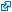#Federal Income Tax Brackets (Tax Year 1922) ARCHIVES

Tax Year 1922 / Filed April 1923

Federal - 1923 Single Tax Brackets
Tax Bracket Tax Rate
\$0.00+ 4%
\$4,000.00+ 8%
\$6,000.00+ 9%
\$10,000.00+ 10%
\$12,000.00+ 11%
\$14,000.00+ 12%
\$16,000.00+ 13%
\$18,000.00+ 14%
\$20,000.00+ 16%
\$22,000.00+ 17%
\$24,000.00+ 18%
\$26,000.00+ 19%
\$28,000.00+ 20%
\$30,000.00+ 21%
\$32,000.00+ 23%
\$36,000.00+ 24%
\$38,000.00+ 25%
\$40,000.00+ 26%
\$42,000.00+ 27%
\$44,000.00+ 28%
\$46,000.00+ 29%
\$48,000.00+ 30%
\$50,000.00+ 31%
\$52,000.00+ 32%
\$54,000.00+ 33%
\$56,000.00+ 34%
\$58,000.00+ 35%
\$60,000.00+ 36%
\$62,000.00+ 37%
\$64,000.00+ 38%
\$66,000.00+ 39%
\$68,000.00+ 40%
\$70,000.00+ 41%
\$72,000.00+ 42%
\$74,000.00+ 43%
\$76,000.00+ 44%
\$78,000.00+ 45%
\$80,000.00+ 46%
\$82,000.00+ 47%
\$84,000.00+ 48%
\$86,000.00+ 49%
\$88,000.00+ 50%
\$90,000.00+ 51%
\$92,000.00+ 52%
\$94,000.00+ 53%
\$96,000.00+ 54%
\$98,000.00+ 55%
\$100,000.00+ 56%
\$150,000.00+ 57%
\$200,000.00+ 58%
Federal - 1923 Married Filing Separately Tax Brackets
Tax Bracket Tax Rate
\$0.00+ 4%
\$4,000.00+ 8%
\$6,000.00+ 9%
\$10,000.00+ 10%
\$12,000.00+ 11%
\$14,000.00+ 12%
\$16,000.00+ 13%
\$18,000.00+ 14%
\$20,000.00+ 16%
\$22,000.00+ 17%
\$24,000.00+ 18%
\$26,000.00+ 19%
\$28,000.00+ 20%
\$30,000.00+ 21%
\$32,000.00+ 23%
\$36,000.00+ 24%
\$38,000.00+ 25%
\$40,000.00+ 26%
\$42,000.00+ 27%
\$44,000.00+ 28%
\$46,000.00+ 29%
\$48,000.00+ 30%
\$50,000.00+ 31%
\$52,000.00+ 32%
\$54,000.00+ 33%
\$56,000.00+ 34%
\$58,000.00+ 35%
\$60,000.00+ 36%
\$62,000.00+ 37%
\$64,000.00+ 38%
\$66,000.00+ 39%
\$68,000.00+ 40%
\$70,000.00+ 41%
\$72,000.00+ 42%
\$74,000.00+ 43%
\$76,000.00+ 44%
\$78,000.00+ 45%
\$80,000.00+ 46%
\$82,000.00+ 47%
\$84,000.00+ 48%
\$86,000.00+ 49%
\$88,000.00+ 50%
\$90,000.00+ 51%
\$92,000.00+ 52%
\$94,000.00+ 53%
\$96,000.00+ 54%
\$98,000.00+ 55%
\$100,000.00+ 56%
\$150,000.00+ 57%
\$200,000.00+ 58%
Federal - 1923 Married Filing Jointly Tax Brackets
Tax Bracket Tax Rate
\$0.00+ 4%
\$4,000.00+ 8%
\$6,000.00+ 9%
\$10,000.00+ 10%
\$12,000.00+ 11%
\$14,000.00+ 12%
\$16,000.00+ 13%
\$18,000.00+ 14%
\$20,000.00+ 16%
\$22,000.00+ 17%
\$24,000.00+ 18%
\$26,000.00+ 19%
\$28,000.00+ 20%
\$30,000.00+ 21%
\$32,000.00+ 23%
\$36,000.00+ 24%
\$38,000.00+ 25%
\$40,000.00+ 26%
\$42,000.00+ 27%
\$44,000.00+ 28%
\$46,000.00+ 29%
\$48,000.00+ 30%
\$50,000.00+ 31%
\$52,000.00+ 32%
\$54,000.00+ 33%
\$56,000.00+ 34%
\$58,000.00+ 35%
\$60,000.00+ 36%
\$62,000.00+ 37%
\$64,000.00+ 38%
\$66,000.00+ 39%
\$68,000.00+ 40%
\$70,000.00+ 41%
\$72,000.00+ 42%
\$74,000.00+ 43%
\$76,000.00+ 44%
\$78,000.00+ 45%
\$80,000.00+ 46%
\$82,000.00+ 47%
\$84,000.00+ 48%
\$86,000.00+ 49%
\$88,000.00+ 50%
\$90,000.00+ 51%
\$92,000.00+ 52%
\$94,000.00+ 53%
\$96,000.00+ 54%
\$98,000.00+ 55%
\$100,000.00+ 56%
\$150,000.00+ 57%
\$200,000.00+ 58%
Federal - 1923 Head of Household Tax Brackets
Tax Bracket Tax Rate
\$0.00+ 4%
\$4,000.00+ 8%
\$6,000.00+ 9%
\$10,000.00+ 10%
\$12,000.00+ 11%
\$14,000.00+ 12%
\$16,000.00+ 13%
\$18,000.00+ 14%
\$20,000.00+ 16%
\$22,000.00+ 17%
\$24,000.00+ 18%
\$26,000.00+ 19%
\$28,000.00+ 20%
\$30,000.00+ 21%
\$32,000.00+ 23%
\$36,000.00+ 24%
\$38,000.00+ 25%
\$40,000.00+ 26%
\$42,000.00+ 27%
\$44,000.00+ 28%
\$46,000.00+ 29%
\$48,000.00+ 30%
\$50,000.00+ 31%
\$52,000.00+ 32%
\$54,000.00+ 33%
\$56,000.00+ 34%
\$58,000.00+ 35%
\$60,000.00+ 36%
\$62,000.00+ 37%
\$64,000.00+ 38%
\$66,000.00+ 39%
\$68,000.00+ 40%
\$70,000.00+ 41%
\$72,000.00+ 42%
\$74,000.00+ 43%
\$76,000.00+ 44%
\$78,000.00+ 45%
\$80,000.00+ 46%
\$82,000.00+ 47%
\$84,000.00+ 48%
\$86,000.00+ 49%
\$88,000.00+ 50%
\$90,000.00+ 51%
\$92,000.00+ 52%
\$94,000.00+ 53%
\$96,000.00+ 54%
\$98,000.00+ 55%
\$100,000.00+ 56%
\$150,000.00+ 57%
\$200,000.00+ 58%

### eFile your Federal tax return noweFiling is easier, faster, and safer than filling out paper tax forms. File your Federal and Federal tax returns online with TurboTax in minutes. FREE for simple returns, with discounts available for Tax-Brackets.org users!

This page shows Tax-Brackets.org's archived Federal tax brackets for tax year 1922. This means that these brackets applied to all income earned in 1922, and the tax return that uses these tax rates was due in April 1923.

Both Federal tax brackets and the associated tax rates were last changed two years prior to 1922 in 1920.

The latest available tax rates are for 2024, and the Federal income tax brackets have been changed since 1922.

### 1923 Federal Tax Deduction Amounts

Tax-Brackets.org also maintains an archive of important Federal income tax exemption amounts, including the Federal Standard Deduction, the Federal Personal Exemption, and the Federal Dependent Deduction. The values of these deductions for tax year 1922 are as follows:

Standard Deduction (Single) Standard Deduction (MFJ) Personal Exemption Dependant Exemption
\$0.00 \$0.00 \$1,000.00 \$400.00

###Sources & Citations

Disclaimer: While we do our best to keep our historical Federal income tax rates up to date and complete, we cannot be held liable for errors or omissions. Is info on this page missing or out-of-date? Please let us know so we can fix it!